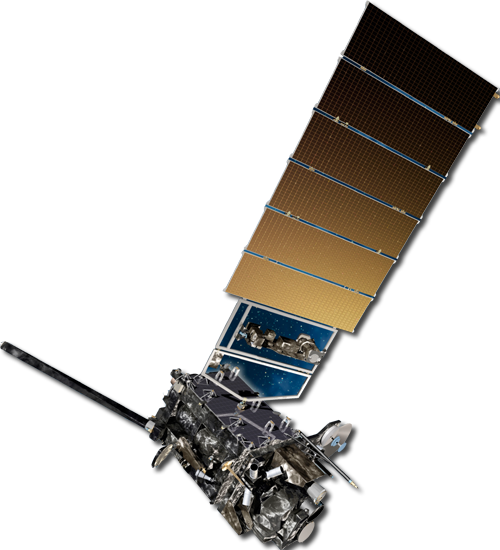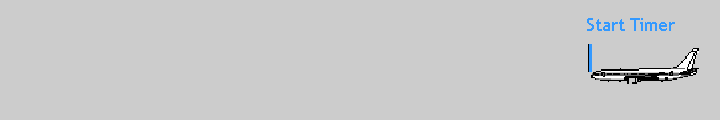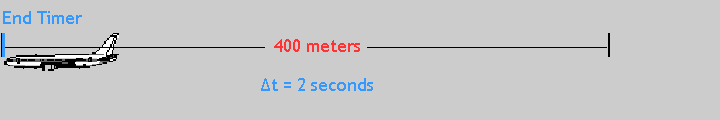6

Satellite Winds# Measuring the Speed of Moving Objects - I

The airplane below is traveling in a straight line at a constant speed. Its speed is the distance traveled divided by the time interval. If we know how far it is traveling in a given time, we can determine its speed.We can calculate this speed if we have two snapshots of the plane separated in time as illustrated by the following two images:The image below was taken two seconds later, after the plane traveled 400 meters.Thus, the airplane speed is 200 meters per second (m/s). Notice the distance is measured from the same spot on the plane, in this example from the nose.

In the above example the plane traveled in a straight line during the time we tracked it. What happens if the object we are tracking does not travel in a straight line?